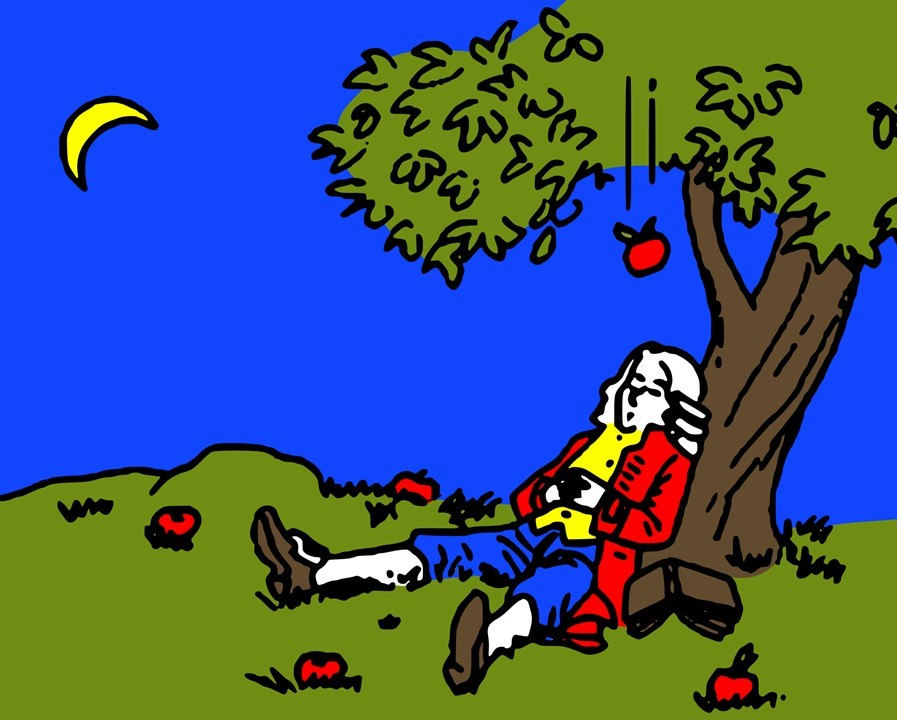# Is gravity a theory or a law?

A lesson on scientific method and scientific terminology.Why is it called Newton's law of gravity and not Newton's theory? Is Einstein's theory of general relativity scientifically inferior to Newton's law? Since in our day-to-day usage, the law carries more weight than the word theory?

Questions like these are doing the rounds on the internet. So we see it fit to explain, once and for all, what scientific terminology is. Before studying science, you must know the meanings of such terms like: axiom, hypothesis, experiment, model, law and theory.

Luckily, this terminology is well incorporated in the method of science. So, let us first understand the scientific method with an example and then we will answer whether gravity is a theory or a law. You will be surprised.

It is observed that bees are attracted to flowers. This statement is taken to be true to serve as a premise or starting point for further reasoning and arguments. This is called an axiom.Bumblebee

Then, a natural question arises. Why are bees attracted to flowers? This is the first step of the scientific method. An observation is followed up by a question. Like, on one summer day, Isaac Newton had questioned the fall of an apple.

One may guess that bees are attracted by the color of flowers. Another person may say that bees are attracted by the nectar inside flowers.

All guesses are called hypotheses.

A scientific hypothesis can be tested in laboratory with the help of an experiment. First, bees are let inside a glass chamber containing artificial flowers. Second, bees are let inside another glass chamber containing real flowers. Further observations are noted down.It is observed that bees are attracted to artificial flowers, they sit on them briefly, then fly away. Thus, the first hypothesis stands true as bees are indeed attracted by the color. In the second set-up, bees sit on real flowers and remain there for long. So, the second hypothesis is also true.

After hypotheses have been tested, it is time to formulate laws, theories or conclusions on basis of the result. In our case, we come to the simple conclusion: "bees are attracted to flowers due to both color and nectar."

A law, on the other hand, is a formula. Like, Newton's law of universal gravitation is used to calculate the "magnitude" of the gravitational force between two objects of mass separated by a given distance.Newton's law

Newton's law is also used in a model so as to mimic remote physical phenomena locally, say on one's computer, such as sky-diving or revolution of Moon around Earth. Thus, a model is generally a simulation. But Newton's law does not attempt to explain how or why gravity works.

In science, theory holds a special place. It is a well-substantiated explanation of the natural world that can incorporate all facts, laws, inferences, and tested hypotheses. So, Einstein's theory of general relativity explains "why" things fall.

While laws rarely change, theories get modified whenever new evidence is discovered. Einstein published his version of the theory in 1915 and since then the theory has adapted as new technologies and new evidence have expanded our view of the universe.Is gravity a theory or a law?

So, is gravity a theory or a law? Well, first of all, it is an always acting force that one can feel. Second, it is both a theory and a law. The law of gravity calculates the amount of attraction while the theory describes why objects attract each other in the first place.

You have now an understanding of terms such as axiom, hypothesis, experiment, law, model and theory, by our use of the scientific method. Whenever you encounter a phrase like "it is just a theory" from the other party, you will know where they lack in their understanding.